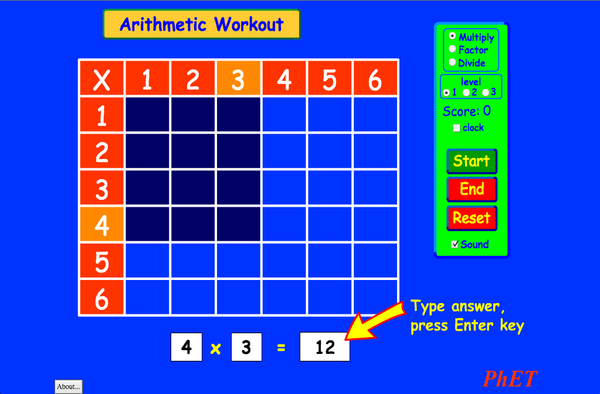АРИТМЕТИКАПревземиВградување затвори Вградете стартувана копија од оваа симулација Користете го ова за да вметнете HTML стартувана копија од оваа симулација. Можете да ја промените ширина и висина на вградената симулација со менување на "ширина"и "висината " атрибутите во HTML. Вградете слика која ќе започне симулацијата со кликање на неа
Кликнете за да работи
Користете го овој HTML код да се прикажат на екранот зборовите "Кликнете за да работи ". Множење PhET е подржан оди едукатори како вас.Back to HTML5 Version

• Множење

Description

Ги запамти ли твоите табели за множење? ... јас не. Подобрете ги вашите вештини за множење, делење и степенување со оваа возбудлива игра. Калкулатори не се дозволени!

Примери за целите на учење

• Објаснете како табелите за множење помагаат да се разбере множењето, степенувањето и делењето.
• Зголемување на точноста во множење, степенување и делење.

Standards Alignment

Common Core - Math

3.OA.A.1
Interpret products of whole numbers, e.g., interpret 5 × 7 as the total number of objects in 5 groups of 7 objects each. For example, describe a context in which a total number of objects can be expressed as 5 × 7.
3.OA.A.2
Interpret whole-number quotients of whole numbers, e.g., interpret 56 ÷ 8 as the number of objects in each share when 56 objects are partitioned equally into 8 shares, or as a number of shares when 56 objects are partitioned into equal shares of 8 objects each. For example, describe a context in which a number of shares or a number of groups can be expressed as 56 ÷ 8.
3.OA.A.4
Determine the unknown whole number in a multiplication or division equation relating three whole numbers. For example, determine the unknown number that makes the equation true in each of the equations 8 × ? = 48, 5 = _ ÷ 3, 6 × 6 = ?
3.OA.B.5
Apply properties of operations as strategies to multiply and divide.2 Examples: If 6 × 4 = 24 is known, then 4 × 6 = 24 is also known. (Commutative property of multiplication.) 3 × 5 × 2 can be found by 3 × 5 = 15, then 15 × 2 = 30, or by 5 × 2 = 10, then 3 × 10 = 30. (Associative property of multiplication.) Knowing that 8 × 5 = 40 and 8 × 2 = 16, one can find 8 × 7 as 8 × (5 + 2) = (8 × 5) + (8 × 2) = 40 + 16 = 56. (Distributive property.)
3.OA.B.6
Understand division as an unknown-factor problem. For example, find 32 ÷ 8 by finding the number that makes 32 when multiplied by 8.
3.OA.C.7
Fluently multiply and divide within 100, using strategies such as the relationship between multiplication and division (e.g., knowing that 8 × 5 = 40, one knows 40 ÷ 5 = 8) or properties of operations. By the end of Grade 3, know from memory all products of two one-digit numbers.
3.OA.D.9
Identify arithmetic patterns (including patterns in the addition table or multiplication table), and explain them using properties of operations. For example, observe that 4 times a number is always even, and explain why 4 times a number can be decomposed into two equal addends.
Version 2.03

Teacher TipsВодичот за наставници (pdf) содржи совети создадени од тимот на PhET.. ( PDF ).

Teacher-Submitted Activities

Arithmetic Games (Inquiry Based)Trish Loeblein ВШ Лаб Математика
Juego de Aritmética (Basado en Indagación)Trish Loeblein, traducido por Diana López СШ
ВШ
Guided
ДомР
Математика
How do PhET simulations fit in my middle school program?Sarah Borenstein СШ Друго Хемија
Географија
Физика
Биологија
MS and HS TEK to Sim Alignment Elyse Zimmer СШ
ВШ
Друго Физика
Биологија
Хемија
Math facts - Using the Multiplication Chart Tapan Sarkar СШ Лаб Математика
Back to the Basics Nyetta Abernathy K-5
СШ
ВШ
Лаб
ДомР
Математика
Discovering divisibility rules Stacy Larson СШ
ВШ
Лаб Математика
곱셈 나눗셈 연습 SIM 사용설명서 이화국(Wha Kuk Lee) СШ
K-5
ДомР
КП
Демо
Физика
Математика
OBJETO DE APRENDIZAGEM: ARITHMETIC Arlete Moura de Oliveira Cabral e Maria Cleide da Silva Barroso K-5 Guided Математика
Operações Aritméticas Fundamentais Georgyana Cidrão; Francisco Régis Vieira Alves K-5 Guided
Лаб
Математика
Албански All shqipAritmetika
Англиски All EnglishArithmetic
Арапски All العربيةarithmetic
Арапски (Саудиска Арабија) All العربية (السعودية)arithmetic
Африкански All AfrikaansRekenkunde
Баскијски All EuskaraAritmetika
Босански All BosanskiARITMETIKA
Бразилски Португалски All português (Brasil)Aritmética
Виетнамски All Tiếng ViệtSố học
Германски All DeutschRechnen
Грузиски All ქართულიარითმეტიკა
Грчки All ΕλληνικάΑριθμητικός
Дански All DanskAritmetisk
Естонски All EestiAritmeetika
Индонезиски All Bahasa IndonesiaAritmatika
Италијански All italianotabelline
Јапонски All 日本語計算
Казахстански All KazakhАрифметика
Кинески - Поедноставен All 中文 (中国)算术
Кинески - Традиционален All 中文 (台灣)算數
Корејски All 한국어곱셈 나눗셈 연습
Латвиски All LatviešuAritmētika
Марати All मराठीअंकगणित
Персиски All فارسیحساب
Полски All polskiArytmetyka
Романски All românăAritmetica
Руски All русскийАрифметика
Свахили All SwahiliHesabu
Словачки All SlovenčinaAritmetika
Српски All СрпскиАРИТМЕТИКА
Туркменистански All TurkmenArifmetika
Турски All TürkçeAritmetik
Унгарски All magyarSzorzótábla játék
Француски All françaisArithmétique
Хебрејски All עבריתחשבון
Холандски All NederlandsRekenen
Хрватски All hrvatskiAritmetika
Шведски All svenskaMatteräkning
Шпански All españolAritmética
Шпански (Мексико) All español (México)Aritmética
Шпански (Перу) All español (Perú)Aritmética
Windows Macintosh Linux
Microsoft Windows
XP/Vista/7/8.1/10
Macromedia Flash 9 or later
OS X 10.9.5 or later
Macromedia Flash 9 or later
Macromedia Flash 9 or later
Развоен Тим Библиотеки од трети лица Благодарност до
• Michael Dubson (developer/lead)
• Trish Loeblein